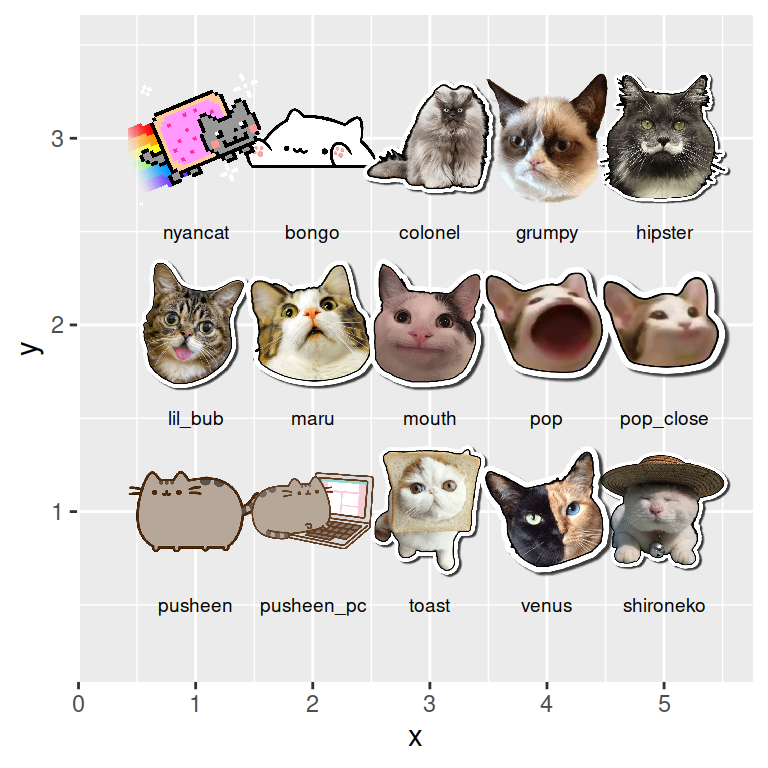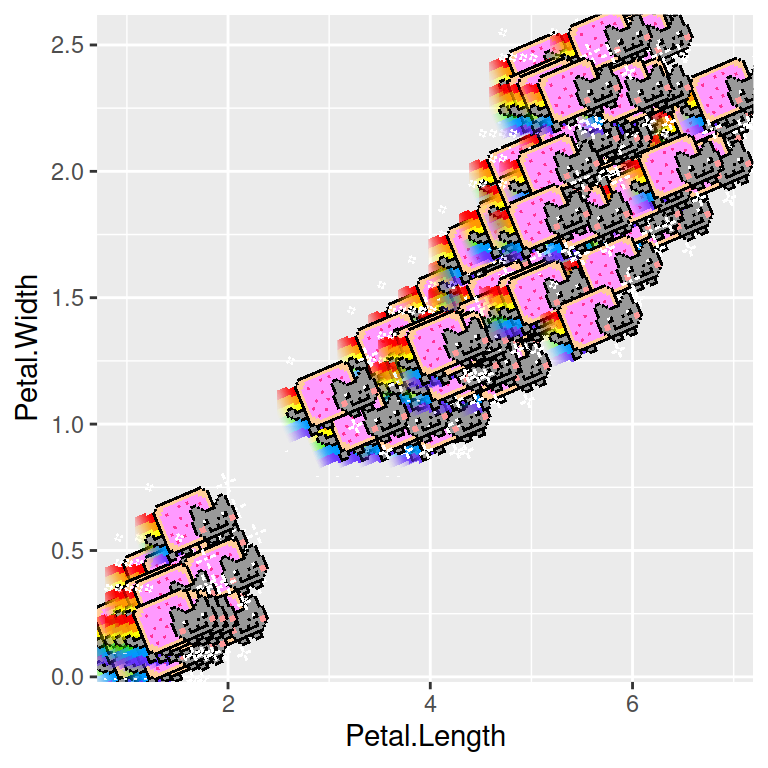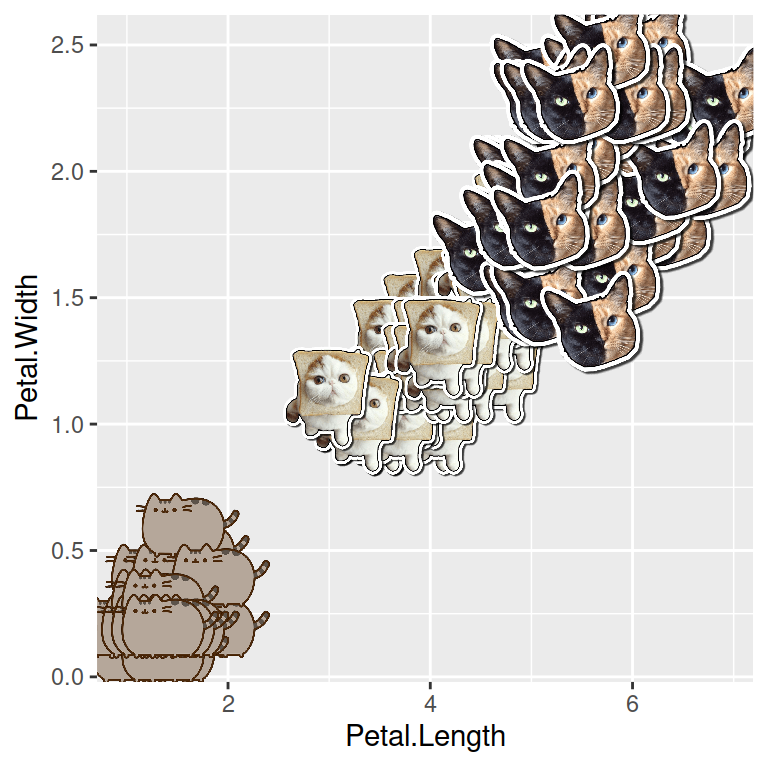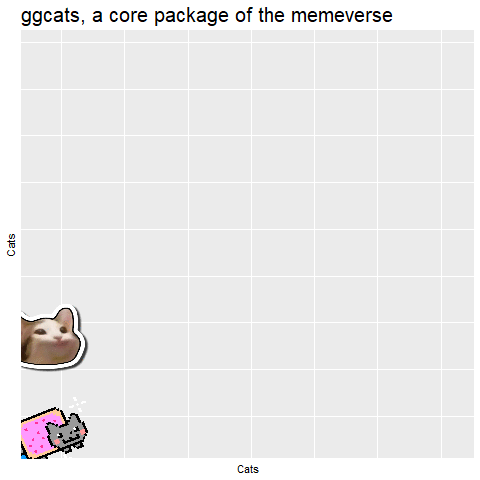# Adding cats to ggplot2 with ggcats

R CODER

## Available cats

The package comes with a geom called `geom_cat` and 15 different PNG files of different popular meme cats to select. These cats are `"nyancat"` (default), `"bongo"`, `"colonel"`, `"grumpy"`, `"hipster"`, `"lil_bub"`, `"maru"`, `"mouth"`, `"pop"`, `"pop_close"`, `"pusheen"`, `"pusheen_pc"`, `"toast"`, `"venus"` and `"shironeko"`. You can see all the cats in the image below.

``````# install.packages("remotes")
# remotes::install_github("R-CoderDotCom/ggcats@main")
library(ggcats)
# install.packages("ggplot2")
library(ggplot2)

grid <- expand.grid(1:5, 3:1)

df <- data.frame(x = grid[, 1],
y = grid[, 2],
image = c("nyancat", "bongo",
"colonel", "grumpy",
"hipster", "lil_bub",
"maru", "mouth",
"pop", "pop_close",
"pusheen", "pusheen_pc",
"toast", "venus",
"shironeko"))

ggplot(df) +
geom_cat(aes(x, y, cat = image), size = 5) +
geom_text(aes(x, y - 0.5, label = image), size = 2.5) +
xlim(c(0.25, 5.5)) +
ylim(c(0.25, 3.5))``````## sCATter plotYou can create a scatter plot the same way as using `geom_point`. Choose a cat with `cat` argument and modify the desired arguments to create your plot.

``````# install.packages("remotes")
# remotes::install_github("R-CoderDotCom/ggcats@main")
library(ggcats)
# install.packages("ggplot2")
library(ggplot2)

# Scatter plot
ggplot(iris, aes(Petal.Length, Petal.Width)) +
geom_cat(cat = "nyancat", size = 4)``````You can also add a different cat based on groups. For that purpose you will need to pass the variable with the cat names to the `cat` argument.

``````# install.packages("remotes")
# remotes::install_github("R-CoderDotCom/ggcats@main")
library(ggcats)
# install.packages("ggplot2")
library(ggplot2)

# Create a new column
iris\$cat <- factor(iris\$Species,
labels = c("pusheen", "toast",
"venus"))

# Scatter plot by group
ggplot(iris, aes(Petal.Length, Petal.Width)) +
geom_cat(aes(cat = cat), size = 4)``````

## Cat animation

An interesting use case for `ggcats` is creating animations. This is specially fun if you combine `"pop"` and `"pop_close"` cats as in the example below.

``````# install.packages("Ecdat")
library(Ecdat)
# install.packages("tidyverse")
library(tidyverse)
# install.packages("gganimate")
library(gganimate)
# install.packages("remotes")
# remotes::install_github("R-CoderDotCom/ggcats@main")
library(ggcats)

# Data frame
dat <-
incomeInequality %>%
select(Year, P99, median) %>%
rename(income_median = median,
income_99percent = P99) %>%
pivot_longer(cols = starts_with("income"),
names_to = "income",
names_prefix = "income_")

# Cats for each line
dat\$cat <- rep(NA, 132)
dat\$cat[which(dat\$income == "median")] <- "nyancat"
dat\$cat[which(dat\$income == "99percent")] <- rep(c("pop_close", "pop"), 33)

# Animation
ggplot(dat, aes(x = Year, y = value, group = income, color = income)) +
geom_line(size = 2) +
ggtitle("ggcats, a core package of the memeverse") +
geom_cat(aes(cat = cat), size = 5) +
xlab("Cats") +
ylab("Cats") +
theme(legend.position = "none",
plot.title = element_text(size = 20),
axis.text = element_blank(),
axis.ticks = element_blank()) +
transition_reveal(Year)``````